# Designing a Simple LanguageBefore we can write a compiler, we need to formally specify the source language. We’re going to walk through the design and specification of a simple language.

The first step in designing a language is to answer some basic questions. (The answers might change as the language design evolves, but you must make best-effort answers before embarking on your language-design journey.) Rather than just telling you what those questions are, let’s actually go through the question and answer process for a simple language.

What (and who) is your language for?
For computing simple arithmetic expressions, that may include variables, and printing them. It’s for folks that just want to do simple arithmetic.
What will you call your language and why?
Ael, derived from Arithmetic Expression Language.
What is an example program in your language (complex enough to show off the structure and many or most of its features)?
let x = 3 * (7 + sqrt 2)  // Declarations introduce variables
let y = abs x - 7
print y * x / 2
print 55.283
x = 1             // Wow, an assignment!
print x

What is the structure (syntax) of your language?
A program is a sequence of statements. Each statement is either a declaration, an assignment, or a print statement (for printing the value of an expression). Expressions are made up of numbers or variable names with the usual arithmetic operators and parentheses, using the classic order of operations, along with sqrt and abs functions. Numbers given in base-ten and are either integers or can have a single decimal point (but no exponent part). Identifiers are letters and digits starting with a letter (valid examples: $x$, $y$, $abc1xqWV$). Whitespace can be used liberally around (but not within) symbols. Comments start with // and extend to the end of the line.
What rules does your language have that you can’t capture structurally?
You cannot use a variable unless it has already been fully assigned in a previous statement. You cannot re-declare a variable.
What is the meaning of programs in your language?
Statements are executed one after the other. Print statements will print the value of the expression to standard output, followed by a newline.

## Language Specification

It is one thing to talk about how your language looks and what programs in your language mean, but it is next-level to specify the structure and the meaning in precise, formal, mathematical, unambiguous, and stunningly beautiful notation. It’s near impossible to capture all the fine details in prose.

Note that we said structure and meaning. You need to define both. The formal terms for these aspects are syntax and semantics. We generally start with the syntax.

### Concrete Syntax

Let’s think through, and sketch out, the structure of programs in our language. We want to specify exactly which sequences of characters comprise well-structured, or well-formed, programs. Such a specification is called the concrete syntax of the language. First, a program will be a sequence of statements:

Program

There will be three kinds of statements: declarations (to bring new variables to life), assignments (to update the value of an existing variable), and print statements:

Statement
Identifiers

An identifier is the computer science word for a name of something you define in your code, like a variable, constant, function, type, parameter, or similar thing. A keyword is a special word in your language, like let or if or while—these words generally do not name things, but sometimes, only in rare cases in some languages, they can. If a keyword is not allowed to be an identifier, we call it a reserved word.
Identifiers vs. Variables

Note how in the declaration rule we used id, but in the assignment rule we used Var. That’s because when declaring we are introducing a new identifier, but when assigning, we want to use an existing variable.

While a statement performs an action (with a side-effect), expressions represent the computation of a value. Expressions can be basic numbers or variables, or they can be made up of operators applied to other expressions. We can put parentheses around any expression, too:

Exp

In larger languages, variables can look complicated like players.name, but Ael is so simple that variables cannot be anything more than just a single identifer:

Var

We should specify the low-level details of our numeric literals and identifiers, too. We could get super-detailed with these, but for now let’s be super simple:

num
id

#### Grammars

The diagrams are a great start, but they are a little vague in places (that “not a keyword” for example), and it’s really, really hard to process diagrams by machine. Text is much more dense then pictures, and more machine-friendly. So now it’s time to write a precise, formal grammar for our language. Jumping right in:

FIRST ATTEMPT, NOT FINAL
Ael {
Program   = Statement+
Statement = let id "=" Exp                  --declare
| Var "=" Exp                     --assign
| print Exp                       --print
Exp       = Exp ("+" | "-" | "*"| "/") Exp  --binary
| ("-" | abs | sqrt) Exp          --unary
| Var
| num
| "(" Exp ")"                     --parens
Var       = id
num       = digit+ ("." digit+)?
let       = "let" ~alnum
print     = "print" ~alnum
abs       = "abs" ~alnum
sqrt      = "sqrt" ~alnum
keyword   = let | print | abs | sqrt
id        = ~keyword letter alnum*
space    += "//" (~"\n" any)* ("\n" | end)  --comment
}


What is this cool notation? It’s Ohm. We’ll cover all of the details later, but study the following to begin:

• A grammar is a sequence of rules that, starting from the first, or start rule, match all and only those strings of characters comprising legally structured programs.
• Rule names beginning with lowercase letters are called lexical rules. Rule names beginning with uppercase letters are called phrase rules, and implicitly have a space* between all of the constructs on their right hand side.
• Certain symbols are predefined: letter (matches any unicode letter), digit (matches any Basic Latin digit "0".."9"), alnum (matches any letter or digit), end (matches when you are at the end of the input), space (matches any Unicode whitespace character), and any (matches any character). The start rule is assumed to have an end at the end, even if not explicitly shown.
• Quotes mean you should handle its characters literally, except for the usual escapes for newline (\n), tab (\t), backslash (\\) and friends, as has become conventional in computer science. Also as per convention: * means “zero or more”, + means “one or more”, ? means zero-or-one (i.e., “optional”), and | means “or”.
• The tilde is a “negative lookahead”: it causes matching to fail if the symbol is matched, but it does not actually advance the matching process (i.e., it doesn’t “consume” any characters: if just checks to see if something is coming up or not).
• The += is used to add a new alternative to an existing rule (i.e., a+=b abbreviates a=a|b.) We’ve used it above to add comments to the language. Anything in the space rule is skipped within phrase rules, so this is a perfect place to add comments!
• The -- annotations are not important for the describing the syntax of the language, but are necessary when using the Ohm library to process the grammar. Details for when they are required can be found in the Ohm Documentation. You can ignore them until you starting using the Ohm tools.
Exercise: Show how the use of ~ in the grammar means that letx = 2 parses as an assignment and not as the declaration let x = 2.
Exercise: Show how the use of ~ in the grammar means that let = 5 is illegal. In other words, how, exactly, are keywords prohibited from being identifiers?

#### Lexical vs. Phrase Rules

The lexical rules, with the exception of the special rule for space, show us how to combine characters into words and punctuation which will call tokens. The phrase rules show us how to combine words and symbols into phrases (where the words and symbols can, if you wish, be surrounded by spacing).

Exercise: Meditate on how profound this is. Have you seen anything like this before? Hint: Are you reading this page in English?

This means it is best to think about the concrete syntax as actually having two distinct parts. The first part (the lexical rules) show us how we can tokenize a stream of characters into a stream of tokens, and the second part (the phrase rules) show us how to parse the token stream into the concrete syntax tree. Here’s an example. The source string:

  print // ⿇🌿
420


is made up of these characters:

SPACE SPACE LATIN SMALL LETTER P LATIN SMALL LETTER R LATIN SMALL LETTER I LATIN SMALL LETTER N LATIN SMALL LETTER T TAB SOLIDUS SOLIDUS SPACE KANGXI RADICAL HEMP HERB LINEFEED DIGIT FOUR DIGIT TWO DIGIT ZERO

When we apply the lexical rules to this character stream, skipping the spaces (and comments), we get the token stream:

print num(420)

So much simpler, right! Next, applying the phrase rules allows us to uncover the concrete syntax tree (also known as the parse tree):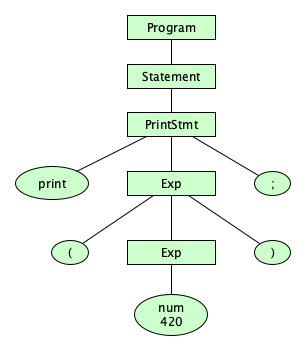A very important thing to note: The frontier of the parse tree is the token stream.

Exercise: Repeat this phrase to yourself five times: The frontier of the parse tree is the token stream.
The parse tree ends at tokens, not characters

Please take the time to consider how silly it would be if the parse tree expanded all of the lexical rules down to characters. Having the parse tree stop at tokens is a good example of what we would call “breaking a complex problem down into simpler parts.”

#### Dealing With Ambiguity

How did we do with our grammar? Well, you know, there is one issue: It can parse the string 9-3*7 in two ways: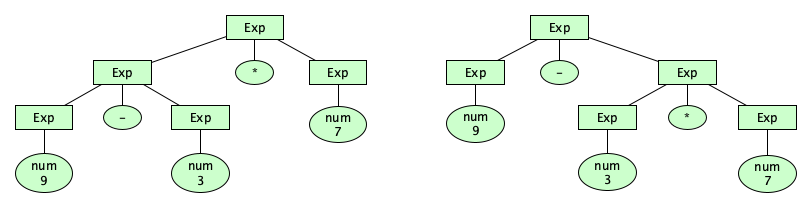Having more than one parse tree for a given input string means that our syntax description is ambiguous. This isn’t necessarily bad, because we could (if we wanted to) leave the ambiguity in the grammar but supply extra informal explanatory text in our language definition that determines how the ambiguity is to be resolved. 🙄🙄🙄 Okay, if you don’t like that idea, we can get rid of this particular ambiguity by adding extra rules in the grammar itself that make an operator precedence emerge:

SECOND ATTEMPT, STILL NOT FINAL
Ael {
Program   = Statement+
Statement = let id "=" Exp                  --declare
| Var "=" Exp                     --assign
| print Exp                       --print
Exp       = Term (("+" | "-") Term)*
Term      = Factor (("*"| "/") Factor)*
Factor    = Var
| num
| ("-" | abs | sqrt) Factor       --unary
| "(" Exp ")"                     --parens
Var       = id
num       = digit+ ("." digit+)?
let       = "let" ~alnum
print     = "print" ~alnum
abs       = "abs" ~alnum
sqrt      = "sqrt" ~alnum
keyword   = let | print | abs | sqrt
id        = ~keyword letter alnum*
space    += "//" (~"\n" any)* ("\n" | end)  --comment
}


Great! Now there is one and only one parse tree for that previously problematic expression: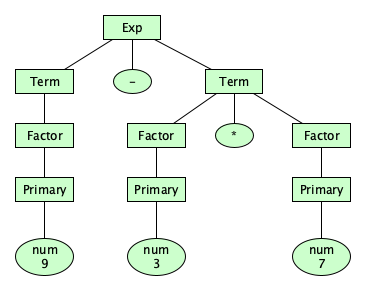Do you see how we made precedence happen? Here’s what we are saying:

• An expression is a sequence of one or more terms, separated by additive operators
• A term is a sequence of one or more factors, separated by multiplicative operators
• A factor is the most basic thing, including parenthesized expressions, which allow unlimited nesting
Exercise: Argue why, or prove if you can that, the new grammar is not ambiguous.

#### Associativity

But we might want to do one more thing. The way things stand now, the parse tree for 3-8-5 looks pretty flat: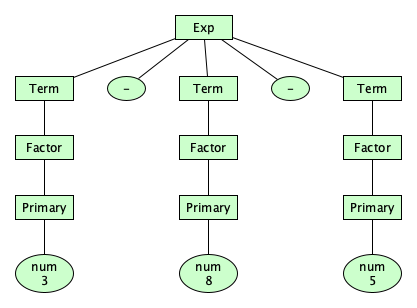It doesn’t suggest whether we mean to compute (3-8)-5 or 3-(8-5). As language designers, we should make the choice of left-associativity or right-associativity for each operator. (Oh, operators can also be non-associative — more on that later.) Let’s make all of our binary operators left-associative:

Ael {
Program   = Statement+
Statement = let id "=" Exp                  --declare
| Var "=" Exp                     --assign
| print Exp                       --print
Exp       = Exp ("+" | "-") Term            --binary
| Term
Term      = Term ("*"| "/") Factor          --binary
| Factor
Factor    = Var
| num
| ("-" | abs | sqrt) Factor       --unary
| "(" Exp ")"                     --parens
Var       = id
num       = digit+ ("." digit+)?
let       = "let" ~alnum
print     = "print" ~alnum
abs       = "abs" ~alnum
sqrt      = "sqrt" ~alnum
keyword   = let | print | abs | sqrt
id        = ~keyword letter alnum*
space    += "//" (~"\n" any)* ("\n" | end)  --comment
}


And we get: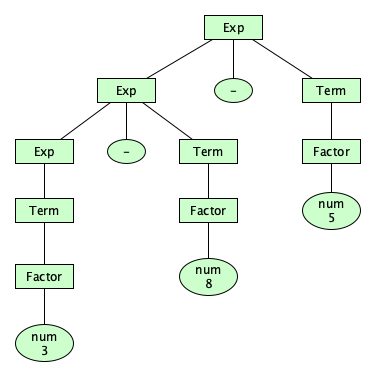#### Testing the Grammar

If you are using Ohm to specify your grammar, you can use the Ohm Editor to experiment with and test your grammar as you write it! We’ll do an in-class demo of it.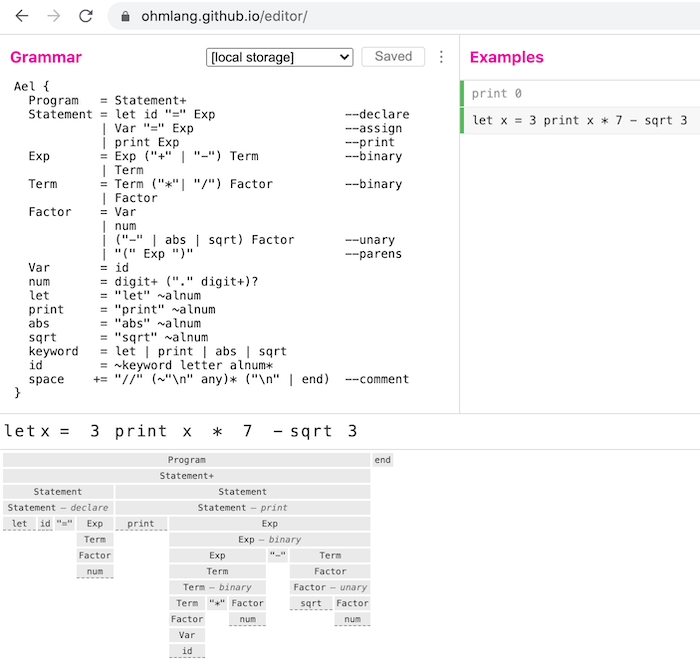CLASSWORK
Get familiar with this tool. Add lots of test cases, both positive and negative.

### Abstract Syntax

We had to do a lot of specification work to define programs as strings of characters. For example, we needed extra tokens (parentheses) and extra categories (Term and Factor) in order to capture precedence, and use other tricks to capture associativity. In order to simplify the remainder of our specification (we haven’t done semantics yet) we need to abstract away this messy stuff. We want to transform concrete syntax trees into simpler (but no less precise!!) abstract syntax trees: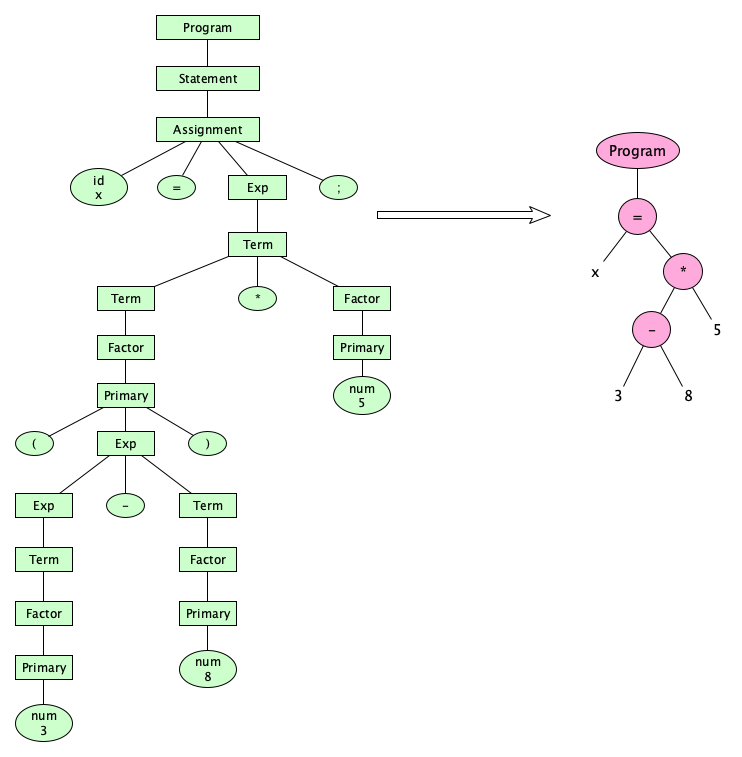Can we formalize this transformation mathematically? Of course we can! Let’s define function $\mathscr{A}$ to turn a parse tree (CST) into the corresponding AST. We write trees in the form $(A\;B\;C\;...)$ where $A$ is the root and $B$, $C$, ... are the children.

$\mathscr{A}[\![S_1 ... S_n]\!] = (\mathsf{Program}\;\mathscr{A}[\![S_1]\!]\;...\;\mathscr{A}[\![S_n]\!])$
$\mathscr{A}[\![\mathrm{let}\;i = E]\!] = (\mathsf{VarDec}\;i\;\mathscr{A}[\![E]\!])$
$\mathscr{A}[\![v = E]\!] = (\mathsf{Assign}\;\mathscr{A}[\![v]\!]\;\mathscr{A}[\![E]\!])$
$\mathscr{A}[\![\mathrm{print}\;E]\!] = (\mathsf{Print}\;\mathscr{A}[\![E]\!])$
$\mathscr{A}[\![E + T]\!] = (\mathsf{Plus}\;\mathscr{A}[\![E]\!]\;\mathscr{A}[\![T]\!])$
$\mathscr{A}[\![E - T]\!] = (\mathsf{Minus}\;\mathscr{A}[\![E]\!]\;\mathscr{A}[\![T]\!])$
$\mathscr{A}[\![T * F]\!] = (\mathsf{Times}\;\mathscr{A}[\![T]\!]\;\mathscr{A}[\![F]\!])$
$\mathscr{A}[\![T\;/\;F]\!] = (\mathsf{Divide}\;\mathscr{A}[\![T]\!]\;\mathscr{A}[\![F]\!])$
$\mathscr{A}[\![-\;F]\!] = (\mathsf{Negate}\;\mathscr{A}[\![F]\!])$
$\mathscr{A}[\![\mathrm{abs}\;F]\!] = (\mathsf{Abs}\;\mathscr{A}[\![F]\!])$
$\mathscr{A}[\![\mathrm{sqrt}\;F]\!] = (\mathsf{Sqrt}\;\mathscr{A}[\![F]\!])$
$\mathscr{A}[\![n]\!] = n$
$\mathscr{A}[\![i]\!] = (\mathsf{IdExp}\;i)$
$\mathscr{A}[\![\mathrm{(}\;E\;\mathrm{)}]\!] = \mathscr{A}[\![E]\!]$

When translating to an abstract syntax, we not only get rid of intermediate syntax categories, but we also drop parentheses, commas, semicolons, brackets, and pretty much all punctuation. Punctuation really only exists to give a “tree structure” to our linear program text.

Exercise: Give the parse tree and the abstract syntax tree for 3-5-8-2*8-9-7-1 according to this grammar and abstract syntax.

### Static Semantics

Note that our syntax did not capture the requirement that “you cannot use an identifier in a statement unless it was previously assigned.” You might want to try to build a grammar to do that, but you will find it notoriously difficult: it is a context-sensitive requirement! Context sensitivity is so hard to capture in a grammar that we specify separate semantic rules to enforce them. Semantic rules that are intended to be checked at compile-time, before the program runs, are called static semantic rules.

In our formal language definition, we capture these rules in a Static Semantic Definition: a function that says, for a given program, whether or not it is semantically correct. Basically we want to think about it like this:

• A program is semantically correct is all of its statements are semantically correct
• A statement is semantically correct if all of its expressions are semantically correct and it is not a declaration statement redeclaring an identifier
• An expression is semantically correct if it does not have any subexpressions that are identifiers which have not been declared.

So all of our statements and expressions have to be analyzed for correctness within a context that holds which identifiers have been previously declared. The analysis of a declaration statement will update the context (if necessary) so all future statements will have it available. Belive it or not, you can capture this requirements formally by defining a mathematical function that says whether or not an AST is semantically okay:

$\mathit{ok}(\mathsf{Program}\;s_1\;\ldots\;s_n) = \mathit{ok}\,[s_1\;\ldots\;s_n]\,\varnothing$
$\mathit{ok}\,[s_1\;\ldots\;s_n]\,c =$
$\;\;\;\;\mathit{if}\;n=0\;\mathit{then}\;\mathit{true}$
$\;\;\;\;\mathit{elsif}\;s_1=(\mathsf{VarDec}\;i\;e)\;\mathit{then}\; \mathit{ok}\;s_1\;c \wedge \mathit{ok}\,[s_2 \ldots s_n]\,(c \cup i)$
$\;\;\;\;\mathit{else}\; \mathit{ok}\;s_1\;c \wedge \mathit{ok}\,[s_2 \ldots s_n]\,c$
$\mathit{ok}(\mathsf{VarDec}\;i\,e)\,c = i \notin c \wedge \mathit{ok}\;e\:c$
$\mathit{ok}(\mathsf{Assign}\;i\,e)\,c = \mathit{ok}\;i\:c \wedge \mathit{ok}\;e\:c$
$\mathit{ok}(\mathsf{Print}\;e)\,c = \mathit{ok}\;e$
$\mathit{ok}(\mathsf{Plus}\;e_1\;e_2)\,c = \mathit{ok}\;e_1\:c \wedge \mathit{ok}\;e_2\:c$
$\mathit{ok}(\mathsf{Minus}\;e_1\;e_2)\,c = \mathit{ok}\;e_1\:c \wedge \mathit{ok}\;e_2\:c$
$\mathit{ok}(\mathsf{Times}\;e_1\;e_2)\,c = \mathit{ok}\;e_1\:c \wedge \mathit{ok}\;e_2\:c$
$\mathit{ok}(\mathsf{Divide}\;e_1\;e_2)\,c = \mathit{ok}\;e_1\:c \wedge \mathit{ok}\;e_2\:c$
$\mathit{ok}(\mathsf{Negate}\;e)\,c = \mathit{ok}\;e\:c$
$\mathit{ok}(n)\,c = \mathit{true}$
$\mathit{ok}(\mathsf{IdExp}\;i)\,c = i \in c$
Syntax vs. Static Semantics

The division between what is syntax (what’s in the grammar) and what is static semantics (checked by custom functions outside the grammar) is sometimes a matter of choice. There are some things that are not too bad to put in the grammar, but they might just be ugly. In this case, feel free to use the semantics.
Exercise: Write a syntax rule for nonnegative integers less than $128$.
Exercise: Write a syntax rule for nonnegative integers less than $2^{63}$. Is this busy work?
Exercise: Think about, a little, without overdoing it, why the notion of “you can only use identifiers that have previously been declared” is impossible to capture in a grammar that allows only one symbol on the left-hand-side of each rule. Could multiple left-hand-side symbols help? Again, don’t think about this too much (unless you have an advanced degree in computer science theory perhaps).

### Dynamic Semantics

A dynamic semantics computes the meaning of an abstract syntax tree. In Ael:

• The meaning of a program is the list of values it outputs
• The meaning of a statement is a function mapping a program state to the next program state. A program state consists of two things: (1) the current values of all assigned variables (the memory), and (2) the list of generated outputs so far.
• The meaning of an expression, given a memory, is the value the expression evaluates to.

We can formally define functions that map abstract syntax trees to their meanings:

$\mathscr{P}(\mathsf{Program}\;s_1\;\ldots\;s_n) = \mathscr{S}s_n( \ldots \mathscr{S}s_1(\{\},\;[\,]) \ldots )$
$\mathscr{S}(\mathsf{Print}\;e)(m,o) = (m, \;\mathit{append}(o,\mathscr{E}e\,m))$
$\mathscr{S}(\mathsf{VarDec}\;i\,e)(m,o) = (\mathit{update}(m,i,\mathscr{E}e\,m),\;o)$
$\mathscr{S}(\mathsf{Assign}\;i\,e)(m,o) = (\mathit{update}(m,\mathscr{E}i\,m,\mathscr{E}e\,m),\;o)$
$\mathscr{E}(\mathsf{Plus}\;e_1\;e_2)\,m = \mathscr{E}e_1 m + \mathscr{E}e_2 m$
$\mathscr{E}(\mathsf{Minus}\;e_1\;e_2)\,m = \mathscr{E}e_1 m - \mathscr{E}e_2 m$
$\mathscr{E}(\mathsf{Times}\;e_1\;e_2)\,m = \mathscr{E}e_1 m \times \mathscr{E}e_2 m$
$\mathscr{E}(\mathsf{Divide}\;e_1\;e_2)\,m = \mathscr{E}e_1 m \div \mathscr{E}e_2 m$
$\mathscr{E}(\mathsf{Negate}\;e)\,m = -\mathscr{E}e\,m$
$\mathscr{E}(n)\,m = n$
$\mathscr{E}(\mathsf{IdExp}\;i)\,m = \mathit{lookup}\,(m, i)$

Things seem to have gotten complicated! So let’s go through a complete example, from character string to program meaning, step-by-step, explaining everything along the way.

## Applying the Specification

Let’s determine the meaning of this string. We’ll walk through everything we did so far:

let yo=8 print
-yo     * (22-  7)// 🎉


The character string is:

LATIN SMALL LETTER L LATIN SMALL LETTER E LATIN SMALL LETTER T SPACE LATIN SMALL LETTER Y LATIN SMALL LETTER O EQUAL SIGN DIGIT EIGHT SPACE LATIN SMALL LETTER P LATIN SMALL LETTER R LATIN SMALL LETTER I LATIN SMALL LETTER N LATIN SMALL LETTER T LINEFEED SPACE SPACE HYPHEN LATIN SMALL LETTER Y LATIN SMALL LETTER O SPACE TAB ASTERISK SPACE LEFTPAREN DIGIT TWO DIGIT TWO HYPHEN SPACE SPACE DIGIT SEVEN RIGHTPAREN SOLIDUS SOLIDUS SPACE PARTY POPPER

We first apply the lexical rules to tokenize the string, remembering to skip the spaces (and comments):

let id(yo) = num(8) print - id(yo) * ( numlit(22) - numlit(7) )

Applying the phrase rules uncovers the concrete syntax tree:The static semantics is applied to the concrete syntax tree to produce the abstract syntax tree: $$\begin{array}{l} ( \mathsf{Program} \\ \;\;\;\; ( \mathsf{VarDec} \; \mathrm{yo} \; 8 ) \\ \;\;\;\; ( \mathsf{Print} \\ \;\;\;\;\;\;\;\; ( \mathsf{Times} \\ \;\;\;\;\;\;\;\;\;\;\;\; ( \mathsf{Negate} \; ( \mathsf{IdExp}\;\mathrm{yo})) \\ \;\;\;\;\;\;\;\;\;\;\;\; ( \mathsf{Minus} \; 22 \; 7) ))) \end{array}$$

which we can read more easily in tree form: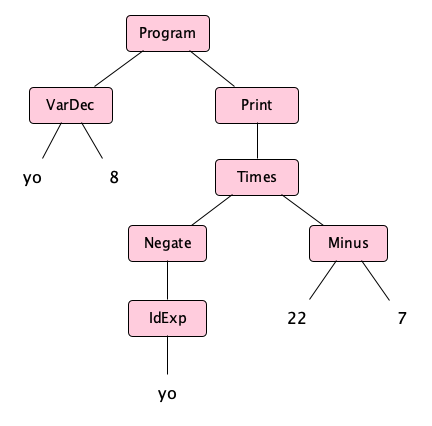which, informally can be shortened to:(The shortened form plays a little loose with the node names, and it elides out the IdExp nodes, because those are, you know, pretty obvious, right?)

Finally, we apply the dynamic semantics to the AST to compute the meaning:

𝒫(Program (VarDec yo 8) (Print (Times (Negate (IdExp yo)) (Minus 22 7)))) =
= (𝒮(Print (Times (Negate (IdExp yo)) (Minus 22 7)))(𝒮(VarDec yo 8)({},[]))) 
= (𝒮(Print (Times (Negate (IdExp yo)) (Minus 22 7)))(𝒮(VarDec yo 8)({},[]))) 
= ({yo:8}, [ℰ(Times (Negate (IdExp yo)) (Minus 22 7)){yo:8}]) 
= [ℰ(Times (Negate (IdExp yo)) (Minus 22 7)){yo:8}]
= [ℰ(Negate (IdExp yo)){yo:8} × ℰ(Minus 22 7){yo:8}]
= [ℰ(Negate 8){yo:8} × (ℰ(22){yo:8} − ℰ(7){yo:8})]
= [-ℰ(8){yo:8} × (22 − 7)]
= [-8 × (22 − 7)]
= [-120]


So the sequence of values the program prints consists of just one value, -120.

DON’T PANIC

You’ve just been introduced to the wonderful field of denotational semantics, and you’ve seen that programming languages can be given a formal, mathematical definition. That does not mean, however, that to write a compiler you have to deeply understand, let alone even use, this stuff! Still, it’s good to be aware of.

Again it’s ok: Real compilers and interpreters do their translations and evaluations in code, not semantic equations. That’s what you’ll do too. Hang in there!
Exercise: Give the token stream and draw the concrete and abstract syntax trees for the Ael expression 98 * (247 - 6) / 3 / 100 - 701.
Exercise: See if you can find any high-level programming languages in which (* (- 8) (- 22 7)) is the actual way to write arithmetic expressions. Hint: several do exist. Why would anyone design a language this way?

## Next Steps

We’ve scratched the surface of what you need to know to formally specify a language. It’s fun, there there’s a lot more to do. Here’s what is coming:

1. We're going to write a complete compiler for the language we have so far. If you are impatient, peek at the finished product.
2. We’ll look at ways to extend the language but adding new features, such as new operators, new statements, etc. These language features will cause us to think hard about tokenization, writing grammars, defining abstract syntax, and so on. Again, we’ve only scratched the surface!
3. We’ll look at issues in the design of very complex languages.

## Summary

We’ve covered:

• Questions that must be answered at the outset of programming language design
• A formal definition for the programming language Ael
• A walkthrough of understanding the design with an example